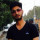Coffee Room
Discuss anything here - everything that you wish to discuss with fellow engineers.
12921 Members
Join this group to post and comment.# Let s and t be two vertices in a undirected graph G=(V,E) having distinct positive edge weights.

Let s and t be two vertices in a undirected graph G=(V,E) having distinct positive edge weights. Let [X,Y] be a partition of V such that s∈X and t∈Y. Consider the edge e having the minimum  weight amongst all those edges that have one vertex in X and one vertex in Y.

Let the weight of an edge e denote the congestion on that edge. The congestion on a path is defined to be the maximum of the congestions on the edges of the path. We wish to find the path from s to t having minimum congestion. Which of the following paths is always such a path of minimum congestion?

1. a path from s to t in the minimum weighted spanning tree

2. a weighted shortest path from s to t

3. an Euler walk from s to t

4. a Hamiltonian path from s to t